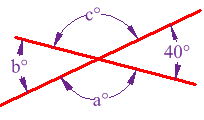# what are vertically opposite angles??

When two lines intersect, four angles are formed at the point of intersection..(∠a and ∠c) and (∠b and ∠d) are called vertically opposite angles.

• 4

what is a transversal line?

• 1

a pair of angles formed by two intersecting lines not having any ray in common are called vertically opposite angles. They have the same measure.

• 4

vertically opposite angles intersect each other and they are equal

• 1

H i !!!!!!!!!!!!!!!

### Example: Find angles a°, b° and c° below:Because b° is vertically opposite 40°, it must also be 40° A full circle is 360°, so that leaves 360° - 2×40° = 280° Angles a° and c° are also vertically opposite, so must be equal, which means they are 140° each. Answer: a = 140°, b = 40° and c = 140°.
• 0

anmol yadav please see that wherever there is ? it is degrees

• 1this is the V O A

• 1

the angle wich is just opposite to its angle

• 1

THE PAIR OF NON-ADJECENT ANGLES FORMED BY THE INTERSECTION OF 2 STRAIGHT LINES ARE CALLED VERTICALLY OPPOSITE ANGLES

• 6

Definition: A pair of non-

formed by the

intersection

of two straight lines

• 0

a pair of angles is said to be vertical (also opposite and vertically opposite, which is abbreviated as vert. opposite ∠s) if the angles are formed from two intersecting lines and the angles are not adjacent..

• 0

those angles which r opp. to each othr

• 0

When two straight lines intersect each other,4 angles are formed (the sum of all these 4 angles is 360 degrees).In such an arrangement a pair of angles is vertically opposite to each other.Such a pair of angles are known as vertically opposite angles.They are always equal.

• 0

If two lines intersect each other, then the pairs of opposite angles so formed are pairs of
vertically opposite angles and they are equal.for example in this figure, as we can see 4 angles r given that area,b,c andd. Out of thesea andc are vertically opp. angles and they are equal same asb andd are vertically opp. angles and they are equal.

Hope it helped!!
Hope it helped u!!

• 0
What are you looking for?